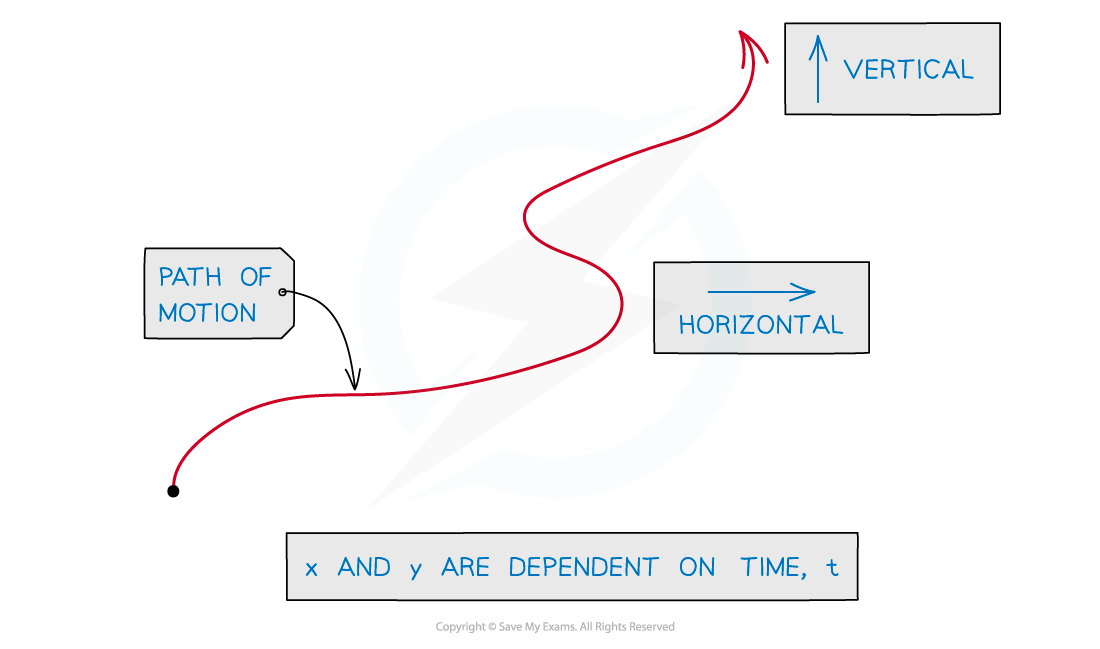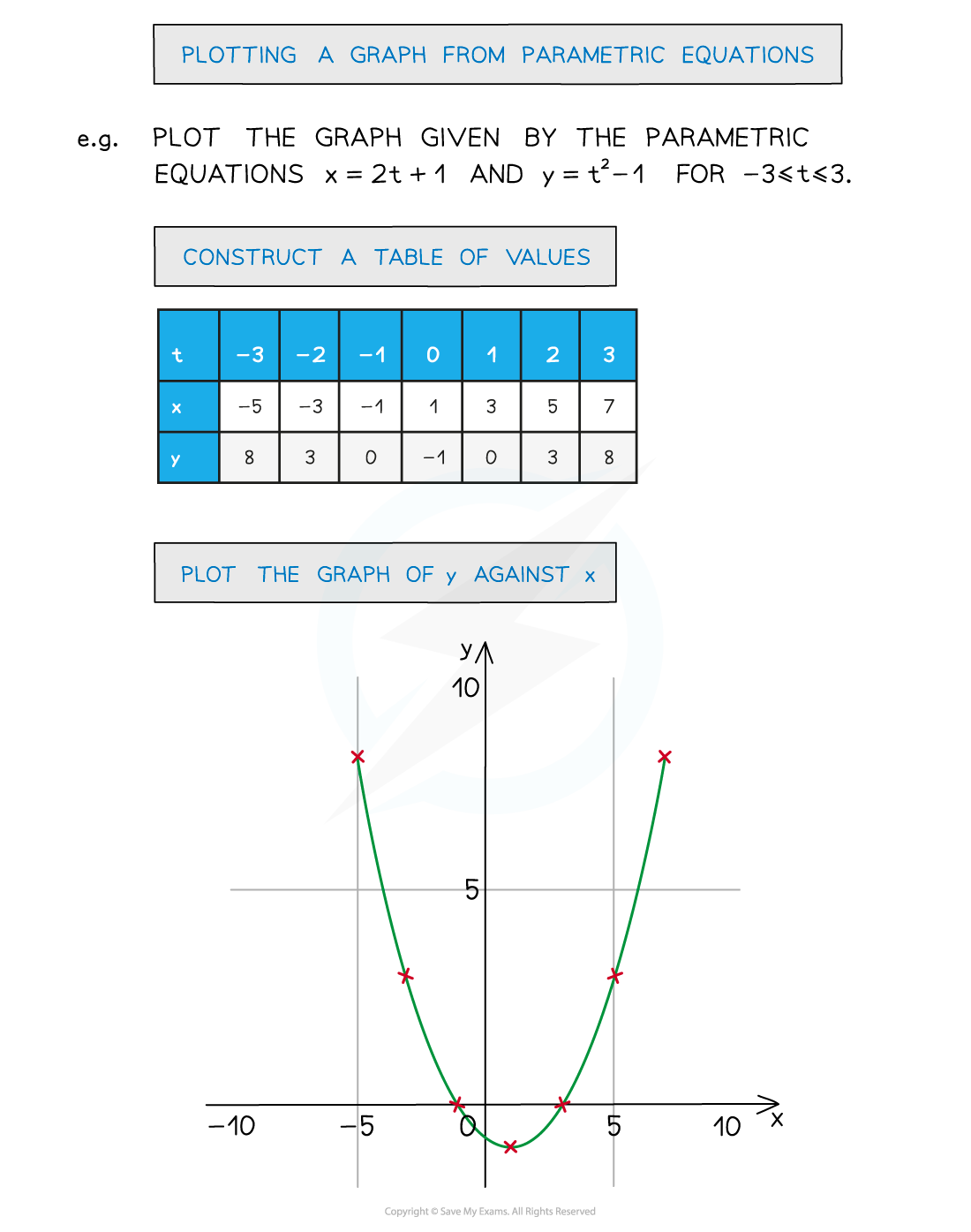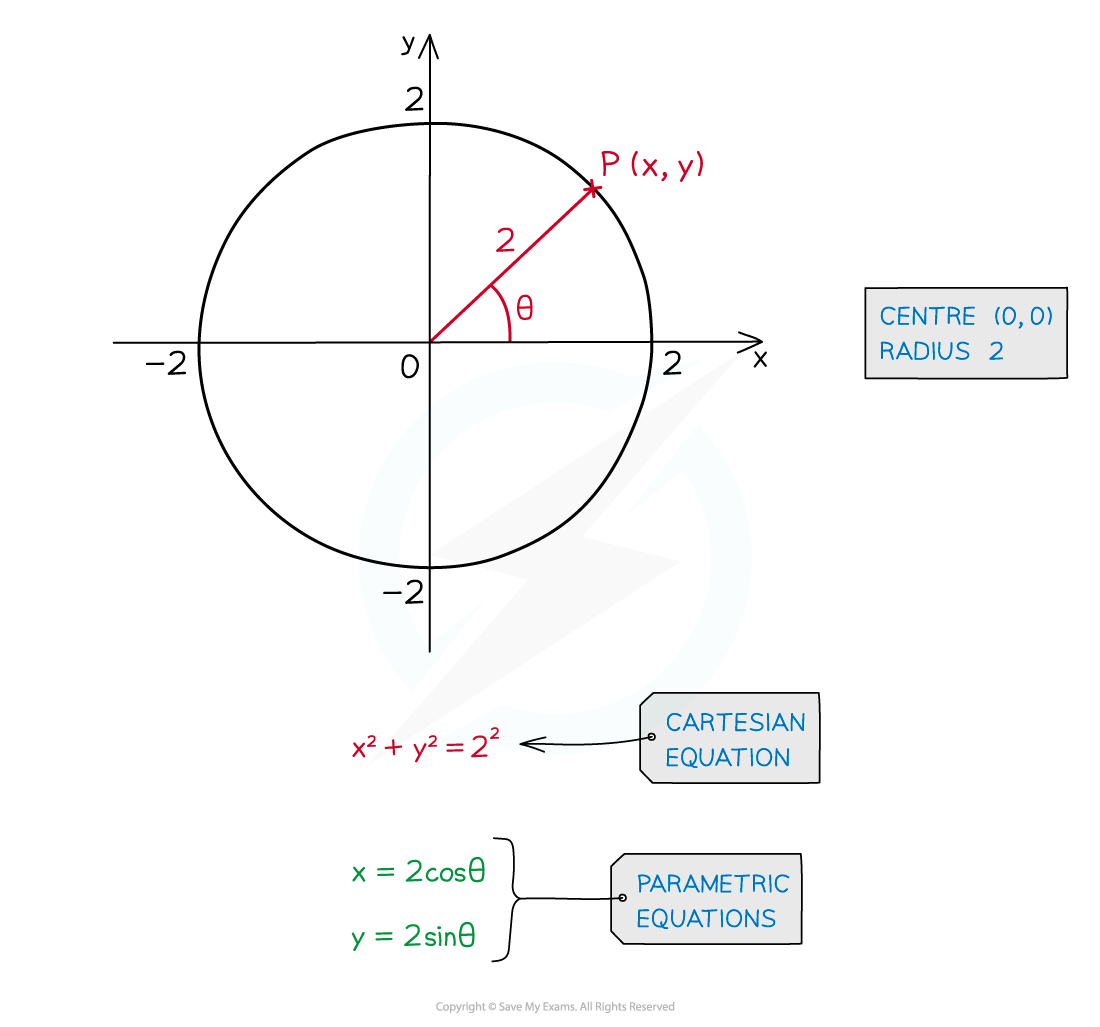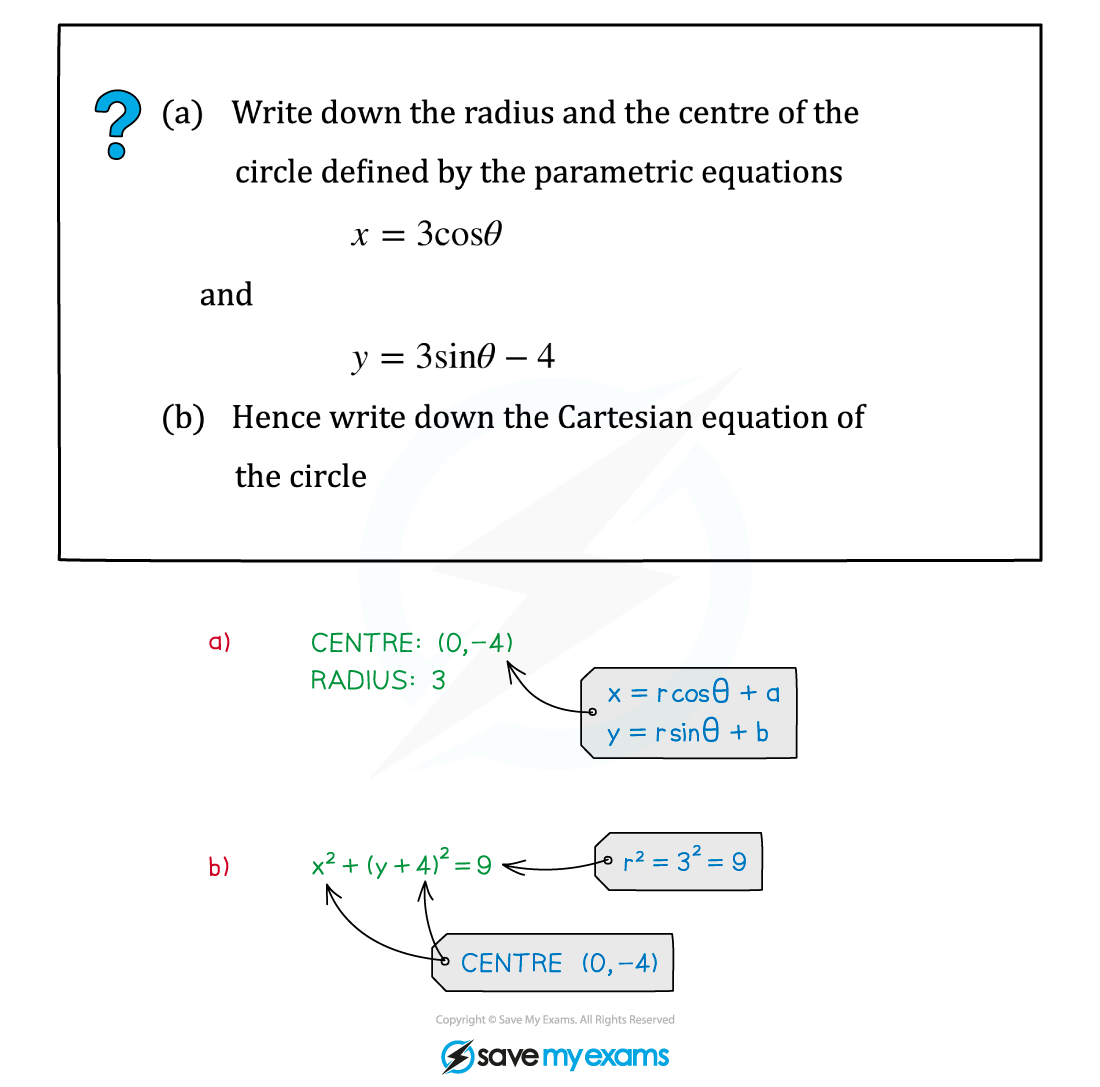# AQA A Level Maths: Pure复习笔记9.1.1 Parametric Equations - Basics

### Parametric Equations - Basics

#### What are parametric equations?

• Graphs are usually described by a Cartesian equation
• The equation involves x and y only
• Equations like this can sometimes be rearranged into the form, y = f(x)• In parametric equations both x and y are dependent on a third variable
• This is called a parameter
• t and θ are often used as parameters
• A common example …
• x is the horizontal position of an object
• y is the vertical position of an object
• and the position of the object is dependent on time t
• x is a function of t, y is a function of t
• x = f(t)
• y = g(t)

#### What do I do with parametric equations?

• It is still possible to plot a graph of y against x from their parametric equations• Also see Parametric Equations – Sketching Graphs

#### What is the link between parametric equations and circles?• For a circle, centre (0, 0) and radius r
• x = rcos θ
• y = rsin θ
• (Note that r is constant, this is not two parameters)
• For a circle, centre (a, b) and radius r
• x = rcos θ + a
• y = rsin θ + b

#### Worked Example